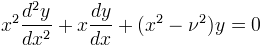Computes both solutions of the Bessel differential equation.

Namespace:  Meta.Numerics.Functions
Assembly:  Meta.Numerics (in Meta.Numerics.dll) Version: 4.1.4Syntax
```public static SolutionPair Bessel(
double nu,
double x
)```

#### Parameters

nu
Type: SystemDouble
The order, which must be non-negative.
x
Type: SystemDouble
The argument, which must be non-negative.

#### Return Value

Type: SolutionPair
The values of Jnu(x), J'nu(x), Ynu(x), and Y'nu(x).Exceptions
ExceptionCondition
ArgumentOutOfRangeExceptionnu or x is negative.Remarks

Bessel functions often occur in the analysis of physical phenomena with cylindrical symmetry. They satisfy the differential equation:Since this is a second order linear equation, it has two linearly independent solutions. The regular Bessel function Jν(x), which is finite at the origin, and the irregular Bessel function Yν(x), which diverges at the origin. Far from the origin, both functions are oscilatory.

This method simultaneously computes both Bessel functions and their derivatives. If you need both J and Y, it is faster to call this method once than to call BesselJ(Double, Double) and BesselY(Double, Double) seperately. If on, the other hand, you need only J or only Y, it is faster to call the appropriate method to compute the one you need.See Also